# Divergence theorem

(diff) ← Older revision | Latest revision (diff) | Newer revision → (diff)

A formula in the integral calculus of functions in several variables that establishes a link between an-fold integral over a domain and an-fold integral over its boundary. Let the functions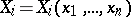and their partial derivatives,, be Lebesgue integrable in a bounded domain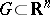whose boundary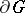is the union of a finite set of piecewise-smooth-dimensional hypersurfaces oriented using the exterior normal. The Ostrogradski formula then takes the form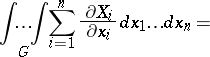(1)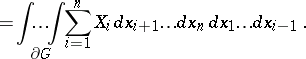If,, are the direction cosines of the exterior normalsof the hypersurfaces forming the boundaryof, then formula (1) can be expressed in the form(2)

whereis the-dimensional volume element inwhileis the-dimensional volume element on.

In terms of the vector field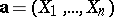, the formulas (1) and (2) signify the equality of the integral of the divergence of this field over the domainto its flux (see Flux of a vector field) over the boundary of: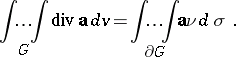For smooth functions, the formula was first obtained for the-dimensional case by M.V. Ostrogradski in 1828 (published in 1831, see ). He later extended it (1834) to cover-fold integrals for an arbitrary natural(published in 1838, see ). Using this formula, Ostrogradski found an expression for the derivative with respect to a parameter of an-fold integral with variable limits, and obtained a formula for the variation of an-fold integral; in one particular case, where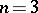, the formula was obtained by C.F. Gauss in 1813, for this reason it is also sometimes called the Ostrogradski–Gauss formula. A generalization of this formula is the Stokes formula for manifolds with boundary.

How to Cite This Entry:
Divergence theorem. Encyclopedia of Mathematics. URL: http://encyclopediaofmath.org/index.php?title=Divergence_theorem&oldid=12667
This article was adapted from an original article by L.D. Kudryavtsev (originator), which appeared in Encyclopedia of Mathematics - ISBN 1402006098. See original article